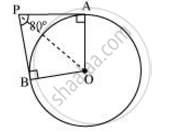Share

Books Shortlist

If tangents PA and PB from a point P to a circle with centre O are inclined to each other an angle of 80°, then ∠POA is equal to - CBSE Class 10 - Mathematics

ConceptNumber of Tangents from a Point on a Circle

Question

If tangents PA and PB from a point P to a circle with centre O are inclined to each other an angle of 80°, then ∠POA is equal to

• 50°

• 60°

• 70°

• 80°

Solution

It is given that PA and PB are tangents.Therefore, the radius drawn to these tangents will be perpendicular to the tangents.

Thus, OA ⊥ PA and OB ⊥ PB

∠OBP = 90º

∠OAP = 90º

In AOBP,

Sum of all interior angles = 360°

∠OAP + ∠APB +∠PBO + ∠BOA = 360°

90° + 80° +90º +∠BOA = 360°

∠BOA = 100°

In ΔOPB and ΔOPA,

AP = BP (Tangents from a point)

OA = OB (Radii of the circle)

OP = OP (Common side)

Therefore, ΔOPB ≅ ΔOPA (SSS congruence criterion)

A ↔ B, P ↔ P, O ↔ O

And thus, ∠POB = ∠POA

anglePOA = 1/2 angleAOB = (100^@)/2 = 50^@

Hence, alternative 50° is correct.

Is there an error in this question or solution?

Video TutorialsVIEW ALL 

Solution If tangents PA and PB from a point P to a circle with centre O are inclined to each other an angle of 80°, then ∠POA is equal to Concept: Number of Tangents from a Point on a Circle.
S